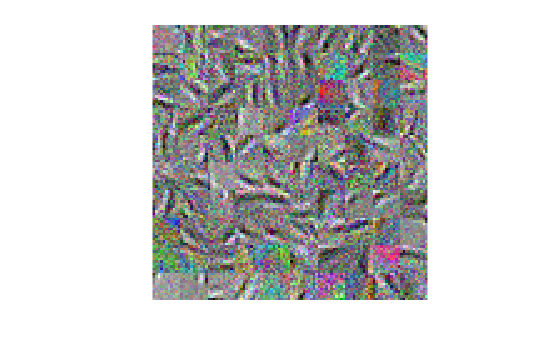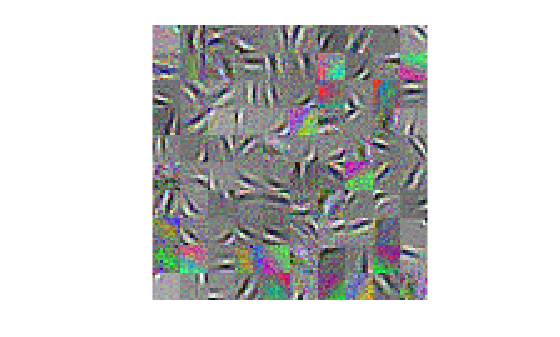sparsefilt

Feature extraction by using sparse filtering

Description

Mdl = sparsefilt(X,q) returns a sparse filtering model object that contains the results from applying sparse filtering to the table or matrix of predictor data X containing p variables. q is the number of features to extract from X, therefore sparsefilt learns a p-by-q matrix of transformation weights. For undercomplete or overcomplete feature representations, q can be less than or greater than the number of predictor variables, respectively.

• To access the learned transformation weights, use Mdl.TransformWeights.

• To transform X to the new set of features by using the learned transformation, pass Mdl and X to transform.

example

Mdl = sparsefilt(X,q,Name,Value) uses additional options specified by one or more Name,Value pair arguments. For example, you can standardize the predictor data or apply L2 regularization.

Examples

collapse all

Create a SparseFiltering object by using the sparsefilt function.

size(data.X)
ans = 1×2

5000         363

There are 5,000 image patches, each containing 363 features.

Extract 100 features from the data.

rng default % For reproducibility
Q = 100;
obj = sparsefilt(data.X,Q,'IterationLimit',100)
Warning: Solver LBFGS was not able to converge to a solution.
obj =
SparseFiltering
ModelParameters: [1x1 struct]
NumPredictors: 363
NumLearnedFeatures: 100
Mu: []
Sigma: []
FitInfo: [1x1 struct]
TransformWeights: [363x100 double]
InitialTransformWeights: []

Properties, Methods

sparsefilt issues a warning because it stopped due to reaching the iteration limit, instead of reaching a step-size limit or a gradient-size limit. You can still use the learned features in the returned object by calling the transform function.

Continue optimizing a sparse filter.

size(data.X)
ans = 1×2

5000         363

There are 5,000 image patches, each containing 363 features.

Extract 100 features from the data and use an iteration limit of 20.

rng default % For reproducibility
q = 100;
Mdl = sparsefilt(data.X,q,'IterationLimit',20);
Warning: Solver LBFGS was not able to converge to a solution.

View the resulting transformation matrix as image patches.

wts = Mdl.TransformWeights;
W = reshape(wts,[11,11,3,q]);
[dx,dy,~,~] = size(W);
for f = 1:q
Wvec = W(:,:,:,f);
Wvec = Wvec(:);
Wvec =(Wvec - min(Wvec))/(max(Wvec) - min(Wvec));
W(:,:,:,f) = reshape(Wvec,dx,dy,3);
end
m   = ceil(sqrt(q));
n   = m;
img = zeros(m*dx,n*dy,3);
f   = 1;
for i = 1:m
for j = 1:n
if (f <= q)
img((i-1)*dx+1:i*dx,(j-1)*dy+1:j*dy,:) = W(:,:,:,f);
f = f+1;
end
end
end
imshow(img,'InitialMagnification',300);The image patches appear noisy. To clean up the noise, try more iterations. Restart the optimization from where it stopped for another 40 iterations.

Mdl = sparsefilt(data.X,q,'IterationLimit',40,'InitialTransformWeights',wts);
Warning: Solver LBFGS was not able to converge to a solution.

View the updated transformation matrix as image patches.

wts = Mdl.TransformWeights;
W = reshape(wts,[11,11,3,q]);
[dx,dy,~,~] = size(W);
for f = 1:q
Wvec = W(:,:,:,f);
Wvec = Wvec(:);
Wvec =(Wvec - min(Wvec))/(max(Wvec) - min(Wvec));
W(:,:,:,f) = reshape(Wvec,dx,dy,3);
end
m   = ceil(sqrt(q));
n   = m;
img = zeros(m*dx,n*dy,3);
f   = 1;
for i = 1:m
for j = 1:n
if (f <= q)
img((i-1)*dx+1:i*dx,(j-1)*dy+1:j*dy,:) = W(:,:,:,f);
f = f+1;
end
end
end
imshow(img,'InitialMagnification',300);These images are less noisy.

Input Arguments

collapse all

Predictor data, specified as an n-by-p numeric matrix or table. Rows correspond to individual observations and columns correspond to individual predictor variables. If X is a table, then all of its variables must be numeric vectors.

Data Types: single | double | table

Number of features to extract from the predictor data, specified as a positive integer.

sparsefilt stores a p-by-q transform weight matrix in Mdl.TransformWeights. Therefore, setting very large values for q can result in greater memory consumption and increased computation time.

Data Types: single | double

Name-Value Arguments

Specify optional comma-separated pairs of Name,Value arguments. Name is the argument name and Value is the corresponding value. Name must appear inside quotes. You can specify several name and value pair arguments in any order as Name1,Value1,...,NameN,ValueN.

Example: 'Standardize',true,'Lambda',1 standardizes the predictor data and applies a penalty of 1 to the transform weight matrix.

Maximum number of iterations, specified as the comma-separated pair consisting of 'IterationLimit' and a positive integer.

Example: 'IterationLimit',1e6

Data Types: single | double

Verbosity level for monitoring algorithm convergence, specified as the comma-separated pair consisting of 'VerbosityLevel' and a value in this table.

ValueDescription
0sparsefilt does not display convergence information at the command line.
Positive integersparsefilt displays convergence information at the command line.

Convergence Information

FUN VALUEObjective function value.
NORM STEPNorm of the iterative step, meaning the distance between the previous point and the current point.
CURVOK means the weak Wolfe condition is satisfied. This condition is a combination of sufficient decrease of the objective function and a curvature condition.
GAMMAInner product of the step times the gradient difference, divided by the inner product of the gradient difference with itself. The gradient difference is the gradient at the current point minus the gradient at the previous point. Gives diagnostic information on the objective function curvature.
ALPHAStep direction multiplier, which differs from 1 when the algorithm performed a line search.
ACCEPTYES means the algorithm found an acceptable step to take.

Example: 'VerbosityLevel',1

Data Types: single | double

L2 regularization coefficient value for the transform weight matrix, specified as the comma-separated pair consisting of 'Lambda' and a positive numeric scalar. If you specify 0, the default, then there is no regularization term in the objective function.

Example: 'Lambda',0.1

Data Types: single | double

Flag to standardize the predictor data, specified as the comma-separated pair consisting of 'Standardize' and true (1) or false (0).

If Standardize is true, then:

• sparsefilt centers and scales each column of the predictor data (X) by the column mean and standard deviation, respectively.

• sparsefilt extracts new features by using the standardized predictor matrix, and stores the predictor variable means and standard deviations in properties Mu and Sigma of Mdl.

Example: 'Standardize',true

Data Types: logical

Transformation weights that initialize optimization, specified as the comma-separated pair consisting of 'InitialTransformWeights' and a p-by-q numeric matrix. p must be the number of columns or variables in X and q is the value of q.

Tip

You can continue optimizing a previously returned transform weight matrix by passing it as an initial value in another call to sparsefilt. The output model object Mdl stores a learned transform weight matrix in the TransformWeights property.

Example: 'InitialTransformWeights',Mdl.TransformWeights

Data Types: single | double

Relative convergence tolerance on gradient norm, specified as the comma-separated pair consisting of 'GradientTolerance' and a positive numeric scalar. This gradient is the gradient of the objective function.

Data Types: single | double

Absolute convergence tolerance on the step size, specified as the comma-separated pair consisting of 'StepTolerance' and a positive numeric scalar.

Example: 'StepTolerance',1e-4

Data Types: single | double

Output Arguments

collapse all

Learned sparse filtering model, returned as a SparseFiltering model object.

To access properties of Mdl, use dot notation. For example:

• To access the learned transform weights, use Mdl.TransformWeights.

• To access the fitting information structure, use Mdl.FitInfo.

To find sparse filtering coefficients for new data, use the transform function.

Algorithms

The sparsefilt function creates a nonlinear transformation of input features to output features. The transformation is based on optimizing an objective function that encourages the representation of each example by as few output features as possible while at the same time keeping the output features equally active across examples.

For details, see Sparse Filtering Algorithm.